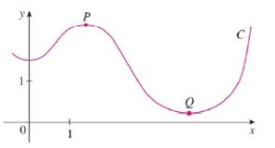Chapter 13.3, Problem 33E

Chapter
Section
Textbook Problem

# (a) Is the curvature of the curve C shown in the figure greater at P or at Q? Explain.(b) Estimate the curvature at P and at Q by sketching the osculating circles at those points.(a)

To determine

To explain: Whether the curvature of curve C is greater at P or Q.

Explanation

The curvature of curve at point is measured in terms of how fast the curve is changing its direction at that particular point. The curve at point P is quickly changing its direction, ...

(b)

To determine

To estimate: The curvature at P or Q using sketch of osculating circles at those points.

### Still sussing out bartleby?

Check out a sample textbook solution.

See a sample solution

#### The Solution to Your Study Problems

Bartleby provides explanations to thousands of textbook problems written by our experts, many with advanced degrees!

Get Started

#### Evaluate the indefinite integral. ex1+exdx

Single Variable Calculus: Early Transcendentals, Volume I

#### In Exercises 2336, find the domain of the function. 32. f(x)=1x2+x2

Applied Calculus for the Managerial, Life, and Social Sciences: A Brief Approach

#### In O,OY=5 and XZ=6. If XWWY, find WZ.

Elementary Geometry for College Students

#### Evaluate . e + 1

Study Guide for Stewart's Single Variable Calculus: Early Transcendentals, 8th

#### Self Check Divide: 3x+19x21

College Algebra (MindTap Course List)